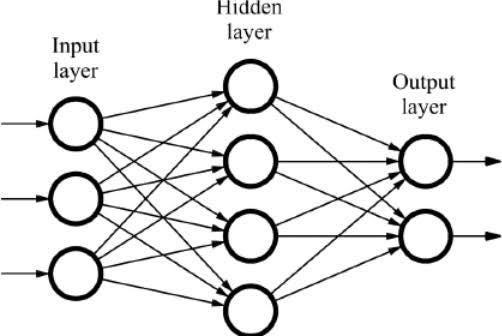# Neural Network

Source: Deep Learning on MediumRegression is used to find the relationship between the variables. This is done by assigning certain weights to the features which affect the target so as to minimize the error.

But in most cases a single regression will not be able to identify the relationships between the variables. Hence, we use a series of regression. This is called neural network.

In simple terms, neural network is basically multiple logistic regression. A series of regression is performed to understand the relationship between the variables. This happens in many layers.

The starting layer is the Input layer and the last layer is the Output layer. All the layers in between are called hidden layers. Each hidden layer is called a perceptron. A neural network can have multiple hidden layers. Therefore, it is also called MLP(Multi Layer Perceptron).

Each point in a layer is called a node. The number of nodes in each layer need not be the same. Each layer learns something from the previous layer. In the above example, layer(2) learns from layer(1), layer(3) learns from layer(2) and so on.

It is advisable to increase the number of hidden layers rather than increasing the number of nodes as it will learn more when there are more layers.

When there are a lot of hidden layers, it is called Deep Learning Neural Network.